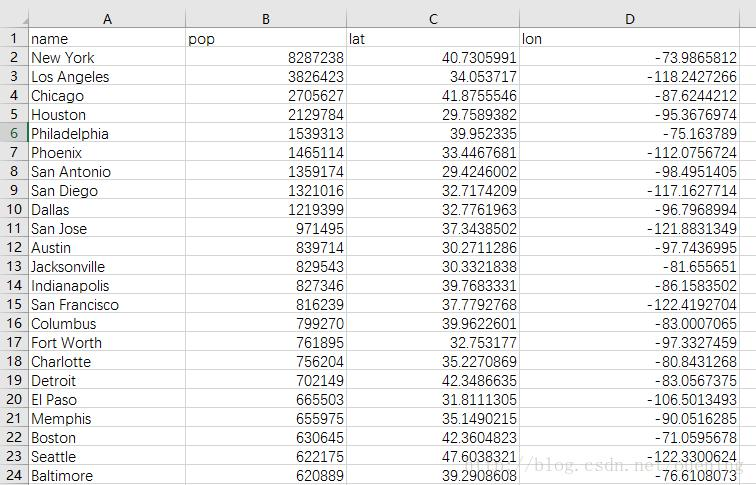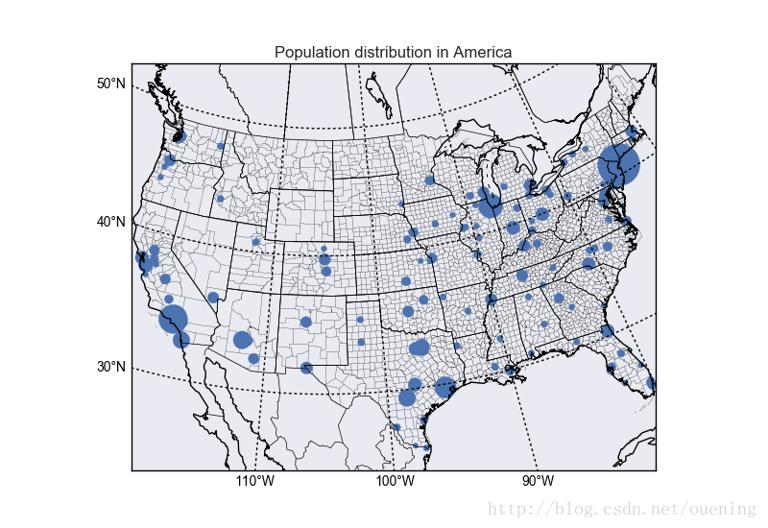# Python/Basemap绘制美国人口分布示意图

## Python/Basemap绘制美国人口分布示意图

2017-10-30 作者: xuzhiping 浏览: 12018 次

Basemap是一个强大的绘制地图工具包，配合matplotlib，可以绘制一些漂亮的地图，通过网上搜集的数据就可以绘制关于人口分布、天气等不同因素在不同地域的分布情况。from mpl_toolkits.basemap import Basemap
import matplotlib.pyplot as plt
import pandas as pd
import numpy as np
from matplotlib import cm

# 绘制基础地图，选择绘制的区域，因为是绘制美国地图，故选取如下经纬度，lat_0和lon_0是地图中心的维度和经度

map = Basemap(projection='stere',lat_0=90,lon_0=-105,\
llcrnrlat=23.41 ,urcrnrlat=45.44,\
llcrnrlon=-118.67,urcrnrlon=-64.52,\
rsphere=6371200.,resolution='l',area_thresh=10000)
# map = Basemap(projection='stere',
#               lat_0=0, lon_0=280,
#               llcrnrlon=73.33,
#               llcrnrlat=3.51,
#               urcrnrlon=112.16,
#               urcrnrlat=53.123)

map.drawmapboundary()   # 绘制边界
#map.fillcontinents()   # 填充大陆，发现填充之后无法显示散点图，应该是被覆盖了
map.drawstates()        # 绘制州
map.drawcoastlines()    # 绘制海岸线
map.drawcountries()     # 绘制国家
map.drawcounties()      # 绘制县

parallels = np.arange(0.,90,10.)
map.drawparallels(parallels,labels=[1,0,0,0],fontsize=10) # 绘制纬线

meridians = np.arange(-110.,-60.,10.)
map.drawmeridians(meridians,labels=[0,0,0,1],fontsize=10) # 绘制经线

## 原始数据有3228组数据，我只选择了180个城市的数据
lat = np.array(posi["lat"][0:180])                        # 获取维度之维度值
lon = np.array(posi["lon"][0:180])                        # 获取经度值
pop = np.array(posi["pop"][0:180],dtype=float)    # 获取人口数，转化为numpy浮点型

size=(pop/np.max(pop))*1000     # 绘制散点图时图形的大小，如果之前pop不转换为浮点型会没有大小不一的效果
x,y = map(lon,lat)

# plt.text(x, y, 'Lagos',fontsize=12,fontweight='bold',
#                     ha='left',va='bottom',color='k')
#
#
# x, y = map(lon, lat)
#
# plt.text(x, y, 'Barcelona',fontsize=12,fontweight='bold',
#                     ha='left',va='center',color='k',
#                     bbox=dict(facecolor='b', alpha=0.2))
#

# plt.scatter(x,y,s=size,cmap=cm.hsv,edgecolors=None,facecolors='c')

# plt.scatter(x,y,s=size,cmap=cm.hsv) # 使用matplotlib的散点图绘制函数
map.scatter(x,y,s=size)     # 也可以使用Basemap的methord本身的scatter
plt.title('Population distribution in America')
plt.show()